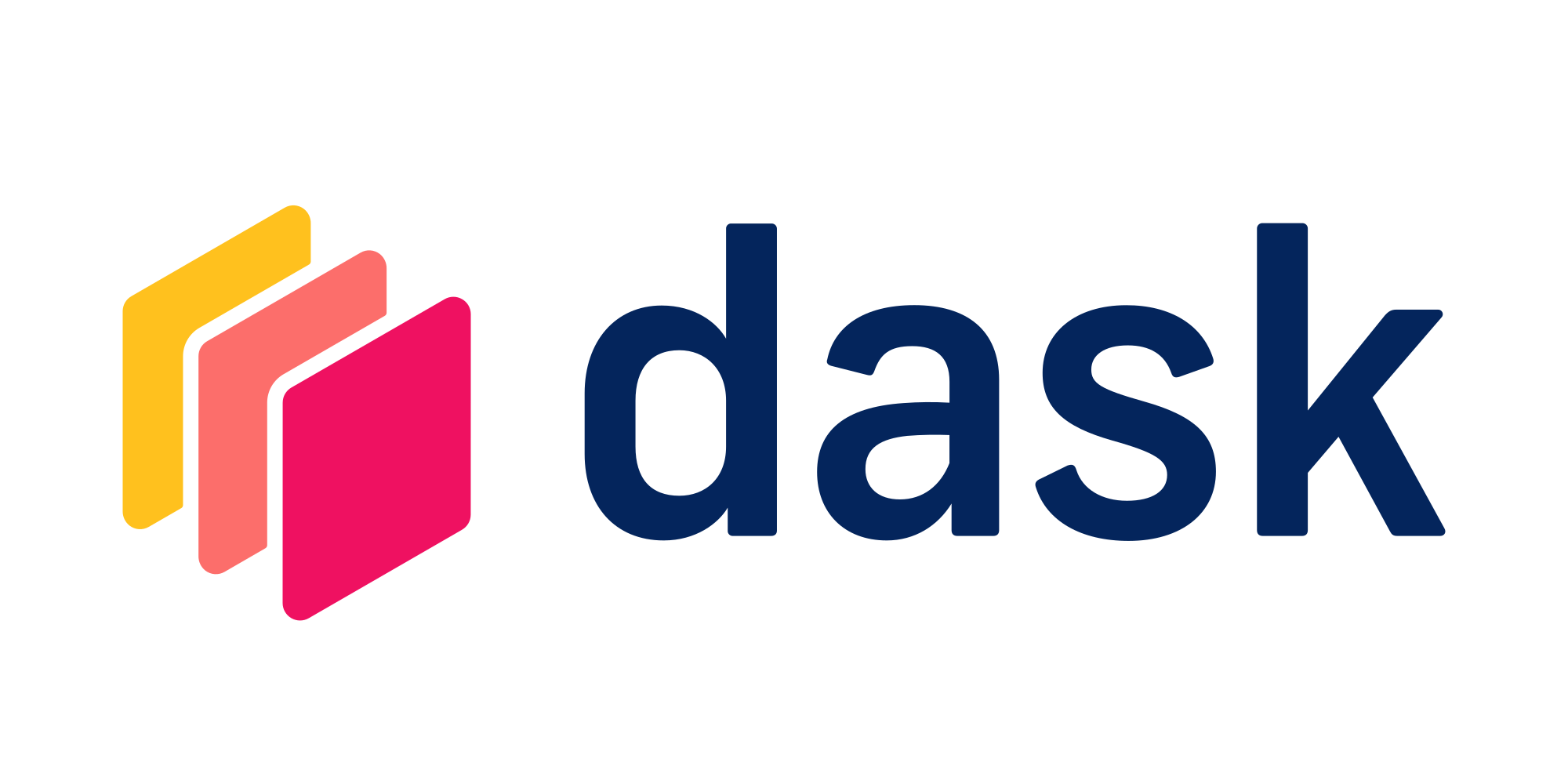# Model Training

Use Dask to train models across a distributed clusterTry this example in seconds on Saturn Cloud

Dask integrates very nicely with existing machine learning libraries like LightGBM, XGBoost, and scikit-learn. Distributing tasks across multiple cores and multiple machines helps in scaling training. In this example, we’ll show how to use Dask when training models from those three libraries.

``````from dask_saturn import SaturnCluster

client = Client(SaturnCluster())
``````

After running the above command, it’s recommended that you check on the Saturn Cloud resource page that the Dask cluster as fully online before continuing. Alternatively, you can use the command `client.wait_for_workers(3)` to halt the notebook execution until all three of the workers are ready.

``````import dask.dataframe as dd

)
``````

We will now assign predictors to X and target feature to y. Dask has a specific module called `dask_ml` that replicates the features of scikit-learn accelerated with parallelization. We will use that feature and split our data to train and test.

``````from dask_ml.model_selection import train_test_split

y = df["SalePrice"]
X = df[["YearBuilt", "BedroomAbvGr"]]

X_train, X_test, y_train, y_test = train_test_split(
X, y, test_size=0.3, shuffle=True, random_state=2
)
``````

LightGBM is a popular algorithm for supervised learning with tabular data. It has been used in many winning solutions in data science competitions. In LightGBM, like in other gradient-boosted decision tree algorithms, trees are built one node at a time. When building a tree, a set of possible “split points” (tuples of (feature, threshold)) is generated, and then each split point is evaluated. The one that leads to the best reduction in loss is chosen and added to the tree. Nodes are added to the tree this way until tree-specific stopping conditions are met.

The `DaskLGBMRegressor` class from `lightgbm` accepts any parameters that can be passed to `lightgbm.LGBRegressor`. Let’s use the default parameters for this example. `lightgbm.dask` model objects also come with a `predict()` method that can be used to create predictions on a Dask Array or Dask DataFrames.

Run the code below to create a validation set and test how well the model we trained in previous steps performs:

``````import lightgbm as lgb

``````

XGBoost is another popular algorithm for supervised learning with tabular data. It’s commonly used by data scientists in many situations, such as the work done at Capital One.

The XGBoost Python package allows for efficient single-machine training using multithreading. However, the amount of training data you can use is limited by the size of that one machine. To solve this problem, XGBoost supports distributed training using several different interfaces. Let us see how distributed training works for XGBoost using Dask.

We will use the test and training set we had created before. `XGBoost` allows you to train on Dask collections like Dask DataFrames and Dask Arrays. This is really powerful because it means that you never have to have a single machine that’s big enough for all of your training data. For more details on this see the XGBoost docs.

Training data for `xgboost.dask` needs to be prepared in a special object called `DaskDMatrix`. This is like the XGBoost DMatrix that you might be familiar with, but is backed by Dask’s distributed collections (Dask DataFrame and Dask Array). The `train()` function from `xgboost` accepts any parameters that can be passed to `xgboost.train()`, with one exception: `nthread`. `xgboost.dask.predict()` can be used to create predictions on a Dask collection using an XGBoost model object.

``````import xgboost as xgb

client=client,
params={
"objective": "reg:squarederror",
"tree_method": "hist",
"learning_rate": 0.1,
"max_depth": 5,
},
dtrain=dtrain,
num_boost_round=50,
)
``````

## Train a Model with scikit-learn and Dask

Many data scientists use scikit-learn as the framework for running machine learning tasks. Conveniently, Dask is intentionally easy to integrate with scikit-learn and has strong API similarities in the `dask-ml` library. In this example, we’ll show you how to create a machine learning pipeline that has all the convenience of scikit-learn but adds the speed and performance of Dask. For more information about dask-ml, visit the official docs.

We’ll train a linear model to predict sale price of houses. We define a Pipeline to encompass both feature scaling and model training. This will be useful in cases like performing a grid search.

``````from sklearn.pipeline import Pipeline

lr = Pipeline(
steps=[
("scale", StandardScaler()),
("clf", LinearRegression(penalty="l2", max_iter=100)),
]
)
``````

Now we are ready to train our model. Before we train, we’ll convert our testing and training sets from dask.dataframe objects to dask.array objects. We’ll also take this chance to precompute the chunksize of our arrays.

``````X_train_arr = X_train.to_dask_array(lengths=True)

lr_fitted = lr.fit(
X_train_arr,
y_train_arr,
)
``````

Evaluate the model against the test set using MSE.

``````from dask_ml.metrics import mean_squared_error

lr_preds = lr_fitted.predict(X_test_arr)
mean_squared_error(y_test_arr, lr_preds, squared=False)
``````

If you want your models to perform even faster, check our example on using RAPIDS on a GPU Cluster, where we have utilized Dask to orchestrate the model training over multiple worker machines, each with NVIDIA GPU to accelerate machine learning workflows.

``````from dask_saturn import SaturnCluster

client = Client(SaturnCluster())

)

y = df["SalePrice"]
X = df[["YearBuilt", "BedroomAbvGr"]]

X_train, X_test, y_train, y_test = train_test_split(
X, y, test_size=0.3, shuffle=True, random_state=2
)

import lightgbm as lgb

import xgboost as xgb

client=client,
params={
"objective": "reg:squarederror",
"tree_method": "hist",
"learning_rate": 0.1,
"max_depth": 5,
},
dtrain=dtrain,
num_boost_round=50,
)

from sklearn.pipeline import Pipeline

lr = Pipeline(
steps=[
("scale", StandardScaler()),
("clf", LinearRegression(penalty="l2", max_iter=100)),
]
)